## 4. What’s the voltage drop if V, = 56 V, V2 = 35 V, and V3 = 3 V O A. 18V O B. 32 V O C. 94 V

Question

4. What’s the voltage drop if V, = 56 V, V2 = 35 V, and V3 = 3 V

O A. 18V

O B. 32 V

O C. 94 V

O D.21 V

in progress 0
5 months 2021-08-18T11:41:11+00:00 2 Answers 4 views 0

The correct option is A. 18 V

Explanation:

The correct option is A. 18 V

Therefore,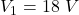Explanation:

Given:

Total Voltage,

V = 56 V,

Voltage drop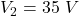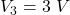To Find: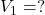(Assumed ,as it is not given clearly in the question)

Solution:

So in series combination total Voltage is given as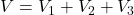Substituting the values we get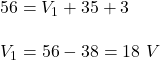Therefore,